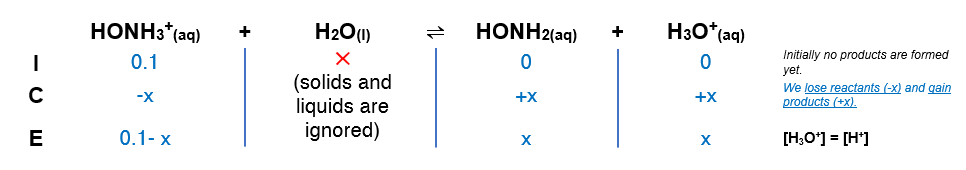# Problem: Calculate the pH of each of the following solutions.b. 0.100 M HONH3Cl

###### FREE Expert Solution

HONH3Cl (aq) → HONH3+ (aq) + Cl- (aq)

Reaction produces an acidic species:

Kb of HONH2 → 9.1x10-9

(1) Setup an ICE chart:(2) Setup Ka expression

$\overline{){{\mathbf{K}}}_{{\mathbf{a}}}{\mathbf{=}}\frac{\mathbf{products}}{\mathbf{reactants}}{\mathbf{=}}\frac{\mathbf{\left[}{\mathbf{H}}_{\mathbf{3}}{\mathbf{O}}^{\mathbf{+}}\mathbf{\right]}\mathbf{\left[}\mathbf{Al}{{\mathbf{\left(}\mathbf{H}}_{}}^{}}{}}$2O)52+][Al(H2O)63+]

Solving for x = [H3O+]

86% (328 ratings)###### Problem Details

Calculate the pH of each of the following solutions.

b. 0.100 M HONH3Cl